# [References] Pyridine is a weak organic base, and readily forms a salt with hydrochloric acid. CsHsN(aq)...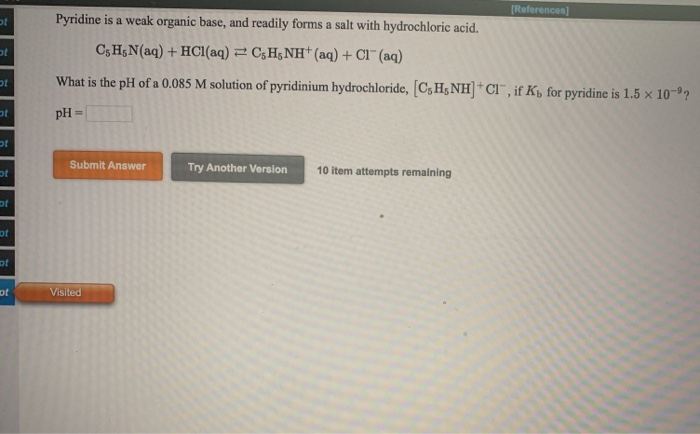[References] Pyridine is a weak organic base, and readily forms a salt with hydrochloric acid. CsHsN(aq) + HCl(aq) = CH NH+ (aq) + Cl" (aq) What is the pH of a 0.085 M solution of pyridinium hydrochloride, CH NH01”, if K, for pyridine is 1.5 x 10-2 pH = Submit Answer Try Another Version 10 item attempts remaining Inila Visited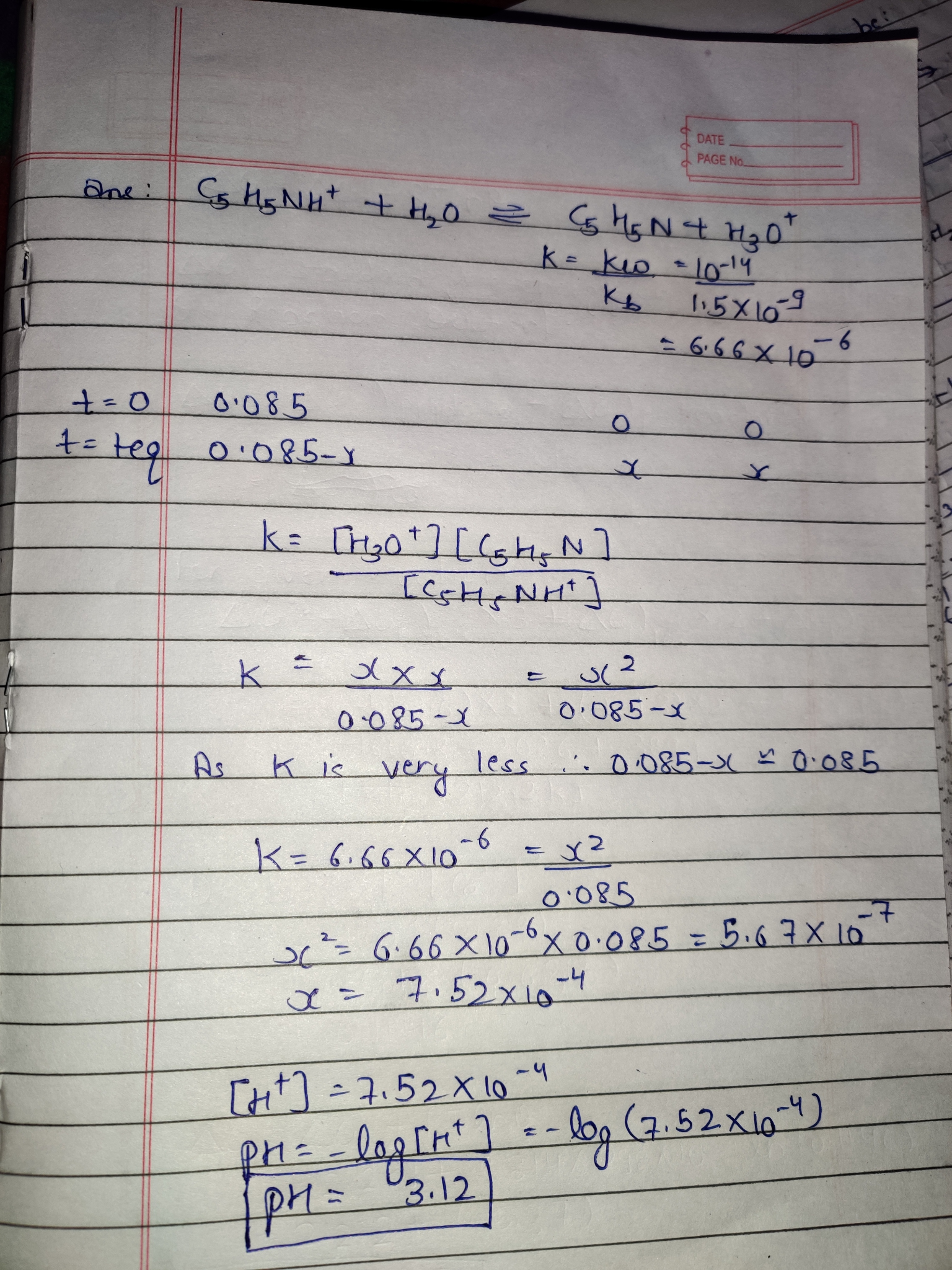#### Earn Coin

Coins can be redeemed for fabulous gifts.

Similar Homework Help Questions
• ### help please Pyridine is a weak organic base, and readily forms a salt with hydrochloric acid....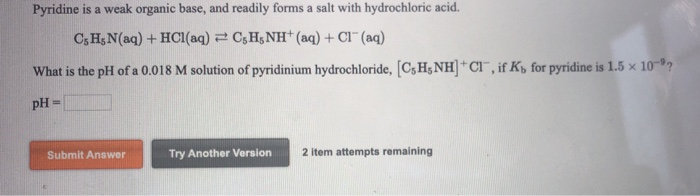help please Pyridine is a weak organic base, and readily forms a salt with hydrochloric acid. CsH; N(aq) + HCl(aq) = CsH NH+ (aq) + CI(aq) CI", if K, for pyridine is 1.5 x What is the pH of a 0.018 M solution of pyridinium hydrochloride, C, H, NH pH = Submit Answer Try Another Version 2 item attempts remaining

• ### 12. Pyridine, C.H.N, is a weak base and reacts with HiCras follows: CH_N (aq) + HCl...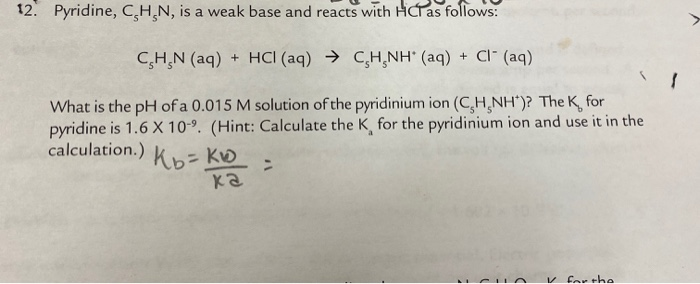12. Pyridine, C.H.N, is a weak base and reacts with HiCras follows: CH_N (aq) + HCl (aq) → CH NH" (aq) + CI+ (aq) What is the pH of a 0.015 M solution of the pyridinium ion (CH NH")? The K for pyridine is 1.6 X 10-9. (Hint: Calculate the K for the pyridinium ion and use it in the calculation.) Khakb ka ch

• ### 1. A hydrogen atom in the organic base pyridine, CsHsN, can be substituted by various atoms...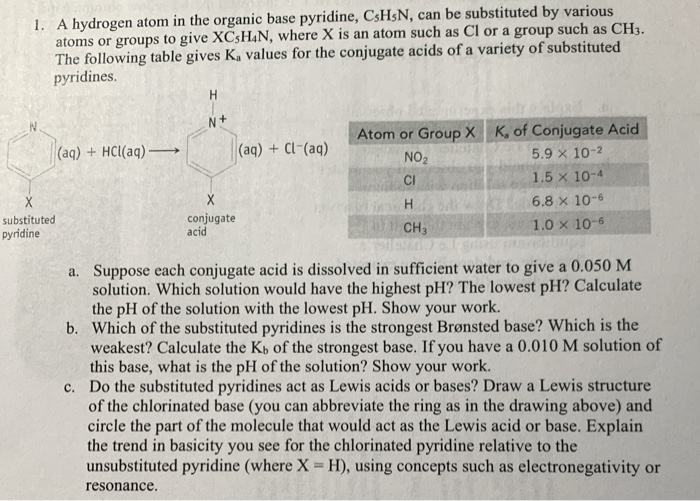1. A hydrogen atom in the organic base pyridine, CsHsN, can be substituted by various atoms or groups to give XCsH4N, where X is an atom such as Cl or a group such as CH3. The following table gives K, values for the conjugate acids of a variety of substituted pyridines. Atom or Group X (aq) + HCl(aq) → (aq) + Cl-(aq) NO K, of Conjugate Acid 5.9 x 10-2 1.5 x 10-4 6.8 x 10-6 1.0 x 10-6 н....

• ### Phenol (CH3OH), commonly called carbolic acid, is a weak organic acid. CH OH(aq) + H20(1) =CH20"...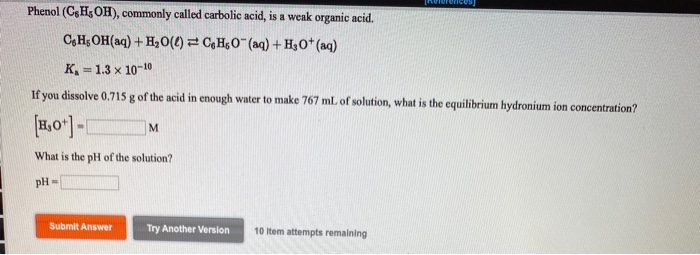Phenol (CH3OH), commonly called carbolic acid, is a weak organic acid. CH OH(aq) + H20(1) =CH20" (aq) + H30+ (aq) K. = 1.3 x 10-10 If you dissolve 0.715 g of the acid in enough water to make 767 mL of solution, what is the equilibrium hydronium ion concentration? (1,0+)-1 What is the pH of the solution? pH- Submit Answer Try Another Version 10 ltem attempts remaining

• ### Phenol (CH3OH), commonly called carbolic acid, is a weak organic acid. CH OH(aq) + H20(1) =CH20"...Phenol (CH3OH), commonly called carbolic acid, is a weak organic acid. CH OH(aq) + H20(1) =CH20" (aq) + H30+ (aq) K. = 1.3 x 10-10 If you dissolve 0.715 g of the acid in enough water to make 767 mL of solution, what is the equilibrium hydronium ion concentration? (1,0+)-1 What is the pH of the solution? pH- Submit Answer Try Another Version 10 ltem attempts remaining

• ### Assignment/takeCovalentActivity.do locator assignment take (References) An 18.6 mL volume of hydrochloric acid reacts completely with a...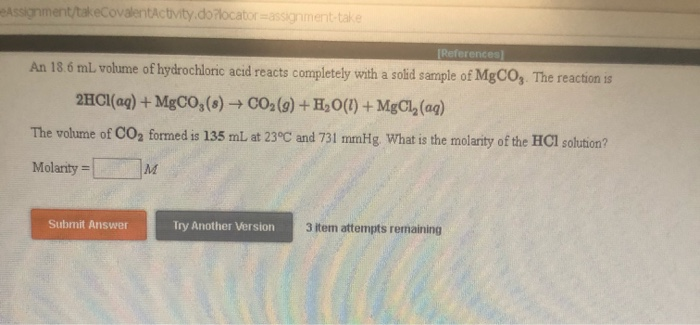Assignment/takeCovalentActivity.do locator assignment take (References) An 18.6 mL volume of hydrochloric acid reacts completely with a solid sample of MgCO,. The reaction is 2HCl(aq) + MgCO3(s) + CO2(g) + H20(1) + MgCl, (aq) The volume of CO2 formed is 135 mL at 23°C and 731 mmHg. What is the molarity of the HCl solution? Molarity = Submit Answer Try Another Version 3 item attempts remaining

• ### What is the general class of each test reaction in the introduction? Precipitation? Acid-Base? Oxidation eduction?...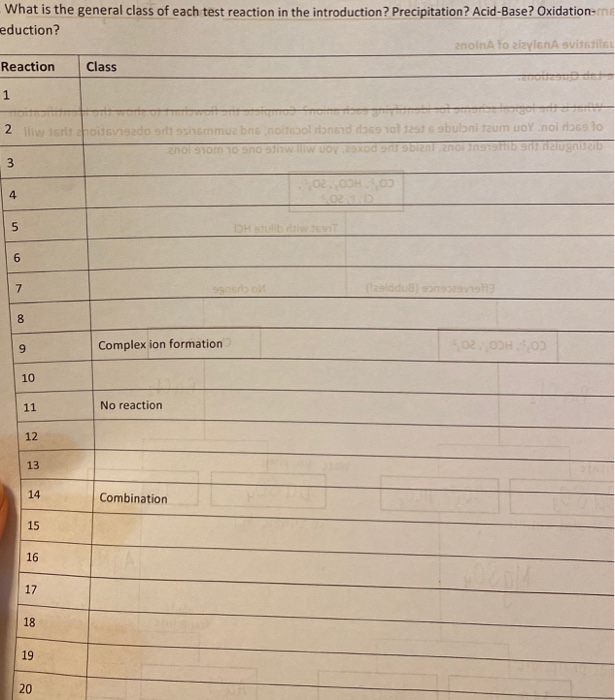What is the general class of each test reaction in the introduction? Precipitation? Acid-Base? Oxidation eduction? anoina to aizy ona svidstils Reaction Class 1 2 iliw 15 TO15192do art sshommua bns nosgol rond dass 101 12575 gbubnium oY noi de lo enor Som 10 910 w II woy Xd on abian aningstid on naloga uzib 3 OOH 4 5 DHET 6 7 SET adus 00 9 Complex ion formation 10 11 No reaction 12 13 14 Combination 15 16 17...

• ### I have a chemistry test that I need help with

I have a chemistry test that I need help with. My answers have stars by them Can someone please help me with the answers to this test??? Part 1 – Matter as Energy & Structure 1. When a pair of electrons is shared between two atoms, a covalent bond is said to have formed. Most often, the pair is formed a. by excess valence electrons b. as one atom contributes both electrons* c. as each atom contributes one electron d....

Free Homework App